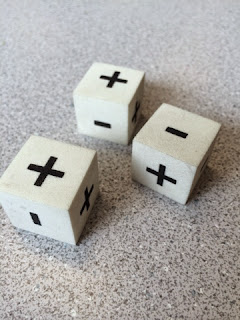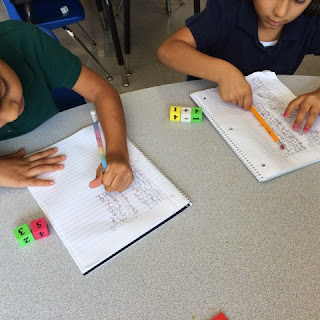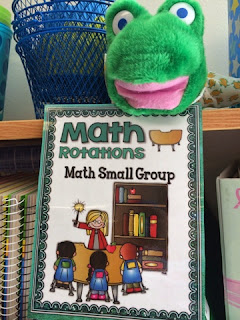One of the math activities that my kids absolutely LOVE is fact practice using addition and subtraction cubes!  This is fact practice-working to memorize math facts.  We do a lot of work with manipulatives composing and decomposing numbers, but we also work to memorize our facts in first grade!
All Levels of Practice
I like the practice because it keeps students at all levels engaged. The cubes I use have four different sets of increasingly difficult numbers to add and subtract.  The cubes were a part of a math manipulative set that was purchased by our district for our classrooms.  I think that they can also be purchased at a teacher supply store.
The green cubes have the numbers 0, 1, 2, 3, 4 and a second 4.
The blue cubes have the numbers 0, 1, 2, 3, 4 and 5.
The yellow cubes have the numbers 1, 2, 3, 4, 5 and 6.
The red cubes have the numbers 4, 5, 6, 7, 8 and 9. The six and the nine are easily different entrusted by a line under the numbers.I also have cubes that have both the plus and minus symbols.
The many possible combinations of cubes give my students a lot of opportunities to master facts up to eighteen.

#### This is how it works:

My student each get two number cubes and an addition/subtraction cube.
Students use their math journals - a spiral-bound notebook that we use for a variety of guided and independent seatwork.

Students roll the cubes and configure them into a number sentence.
They transfer the addition or subtraction fact and create an equation in their journals.  They know to always place the larger number first when the subtraction symbol comes up. (My first graders aren't dealing with negative numbers just yet!)

Students who need more practice with lower numbers begin with the green or yellow cubes.
More advanced students may practice with the yellow and red cubes.
For students who who still need more practice counting to add and subtract, I created cubes of the same colors with pips. Students can count the pips to make addition and subtraction concreate for them.
I also use the same cubes for guided practice during small group math time at my table.I have created these math rotation mini-posters that I use during math workshop/guided math.
You may purchase them from my TpT store here:
More ways to differentiate your math practice:
Try this FREEBIE:
Happy Teaching!

#### 1 comment

1.I love how your dice are color coded. I find that dice are one of the best ways to promote additive reasoning in K-6.

Tara
The Math Maniac